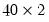Command Reference : Matrix Language Reference

 mtos
Convert matrix to a series or group. Fills a series or group with the data from a vector or matrix.
Syntax
Vector Proc: mtos(vector, series[, sample])
Matrix Proc: mtos(matrix, group[, sample, prefix])
Matrix Proc: mtos(matrix, group[, prefix])
Matrix-TO-Series Object. Include the vector or matrix name in parentheses, followed by a comma and then the series or group name. The number of included observations in the sample must match the row size of the matrix to be converted. If no sample is provided, the matrix is written into the series using the current workfile sample.
For the matrix proc, the prefix parameter is a string. If the target group object does not exist, the group is created and populated with series named <prefix>1, <prefix>2, etc. If the prefix is omitted, the default prefix is “SER”.
Examples
mtos(mom,gr1)
converts the first column of the matrix MOM to the first series in the group GR1, the second column of MOM to the second series in GR1, and so on. The current workfile sample length must match the row length of the matrix MOM. If GR1 is an existing group object, the number of series in GR1 must match the number of columns of MOM. If a group object named GR1 does not exist, EViews creates GR1 with the first series named SER1, the second series named SER2, and so on.
series col1
series col2
group g1 col1 col2
sample s1 1951 1990
mtos(m1,g1,s1)
The first two lines declare series objects, the third line declares a group object, the fourth line declares a sample object, and the fifth line converts the columns of the matrix M1 to series in group G1 using sample S1. This command will generate an error if M1 is not amatrix.
Cross-references
See “Matrix Language”for further discussion and examples of the use of matrices.